• Save

# Numbers Problems with Solutions for SSC and Banking Exams

4 months ago 1.3K Views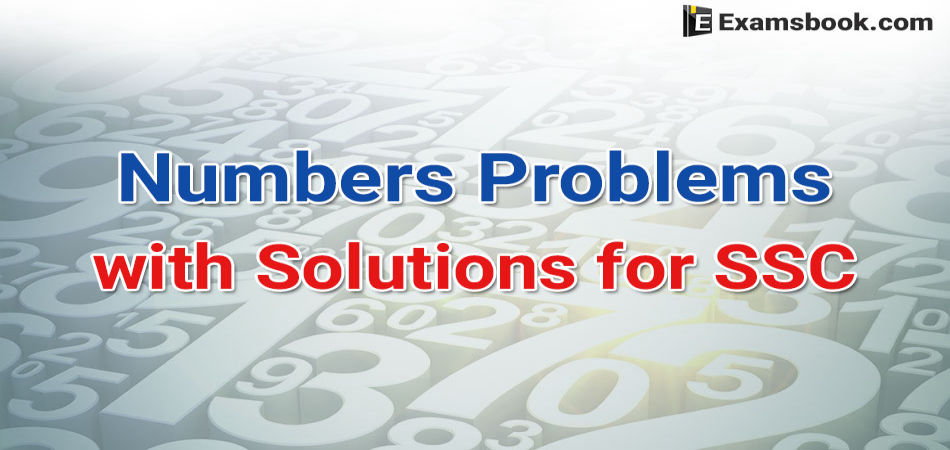Many students are puzzled about the numbers problems in their exams. Problems are part of our life but every problem has a solution. If you are preparing for SSC exam, here you can learn the numbers problems with solutions for SSC and banking exam.

In this blog, you will understand how to face or solve numbers problems which will be beneficial for your upcoming competitive exams. After learning the use of numbers problems with solutions you can practice problem on number aptitude question and answers.

You can read Number System Questions in Hindi if you want to prepare in Hindi.

## Numbers Problems with Solutions for Competitive Exams

Q.1. A number is as much greater than 36 as is less than 86. Find the number.

Solution. Let the number be x. Then, x – 36 = 86 – x ⟺ 2x = 86 + 36 = 122 ⟺ x = 61.

Hence, the required number is 61.

Q.2. The Sum of a rational number and its reciprocal is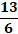. Find the number.

Solution. Let the number be x.

Then,

$${x} + \frac{1}{x} = \frac{13}{6} ⟺\frac{x^2+1}x=\frac{13}{6}⟺6x^2-13x+6 = 0$$

$$⟺ 6x^2-9x-4x+6=0⟺(3x-2)(2x-3)=0$$

$$⟺x=\frac{2}{3} or\frac{3}{2}$$

Hence the required number is $$⟺x=\frac{2}{3} or\frac{3}{2}$$

Q.3. The difference of two numbers is 11 and one-fifth of their sum is 9. Find the numbers.

Solution. Let the number be x and y. Then.

x – y = 11            …(i)

and $$\frac{1}{5} (x + y)= 9 ⟺ x + y = 45$$…….(ii)

Adding (i) and (ii), We get : 2x = 56 or x = 28. Putting x = 28 in (i), we get : y = 17.

Hence, the numbers are 28 and 17.

Q.4. The average of four consecutive even numbers is 27. Find the largest of these numbers.

Solution. Let the four consecutive even number be x, x+2, x+4 and x +6

Then, the sum of these numbers = (27 x 4) = 108.

So, x + (x +2) + (x+4) + (x+6) = 108 or 4x = 96 or x = 24.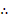Largest number = (x + 6) = 30.

Q.5. Of two numbers, 4 times the smaller one is less than 3 times the larger one by 5. If the sum of the numbers is larger than 6 times their difference by 6, find the two numbers.

Solution. Let the number be x and y, such that x > y.

Then, 3x – 4y = 5 ……….(i)

and (x + y) – 6 ( x – y ) = 6 ⟺ - 5x + 7y = 6………(ii)

Solving (i) and (ii), we get : x = 59 and y = 43.

Hence, the required numbers are 59 and 43.

Q.6. Find a number such that when 15 is subtracted from 7 time the number, the result is 10 more than twice the number.

Solution. Let the number be x. Then, 7x – 15 = 2x + 10 ⟺ 5x = 25 ⟺ x = 5.

Hence, the required number is 5

Q.7. The sum of number is 184. If one-third of the one exceeds one-seventh of the other by 8, find the smaller number.

Solution. Let the numbers be x and (184-x). Then,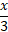-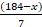= 8  7x – 3(184-x) = 168 10x = 720  x = 72.

So, the numbers are 72 and 112. Hence, smaller number = 72.

Q.8. The sum of two number is 15 and the sum of their squares is 113. Find the numbers.

Solution. Let the numbers be x and (15 – x).

Then,  x2 + (15 – x)2 = 113 ⟺ x2 + 225 + x– 30x = 113

⟺ 2x2 – 30x + 112 = 0  ⟺ x2 – 15x + 56 = 0

⟺ (x – 7) (x – 8) = 0 ⟺ x = 7 or x = 8.

So, the numbers are 7 and 8.

Q.9 The sum of the squares of three consecutive odd numbers is 2531. Find the numbers.

Solution. Let the number be x, x+2 and x+4.

Then, x2 + (x+2)2+(x+4)2= 2531 ⟺ 3x2 + 12x – 2511 = 0

⟺ x2 + 4x – 837 = 0 ⟺ ( x – 27) (x +31) = 0 ⟺ x = 27.

Hence, the required numbers are 27, 29 and 31.

Q.10. The ratio between a two-digit number and the sum of the digit of that number is 4 : 1. If the digit in the unit’s place is 3 more than the digit in the ten’s place, what is the number.

Solution. Let the ten’s digit be x. Then, unit’s digit = (x+3).

Sum of the digits = x + (x +3) = 2x + 3. Number = 10x + (x+3) = 11x + 3.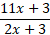=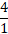⟺ 11x+3 = 4(2x+3)  3x = 9  x = 3.

Hence, required number = 11x + 3 = 36.

I hope these given number problems with solutions help you to solve number problems quickly and correctly in competitive exams. Practice more problems on numbers question and answers.

You can ask me in the comment section if you are facing problems related to number problems with solutions and its use.

All the best for Exams.